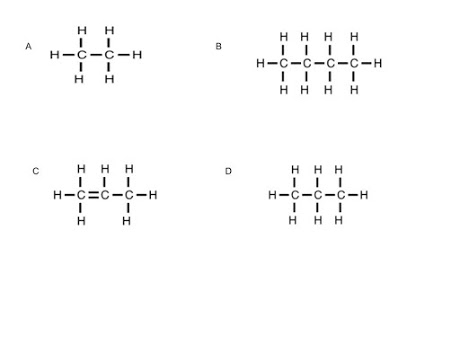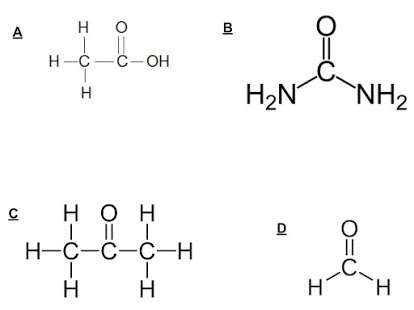Carbon chem review Q 2019
Email *
Last Name *
First Name *
Period *
1. What is the maximum number of Covalent bonds than an atom of oxygen can form?
2. Which hydrocarbon below would contain (1) one double bond and still be built correctly?
3. Refer to diagram 2 below. Which of the following molecules ARE built correctly?
diagram 24. C4H6 could be the the molecular formula for: check all that apply
5. Which of the following molecules below would "behave" like an acid?
diagram 56. What is the correct dot diagram for Carbon?
7. Which chemist was responsible for creating the idea of dot diagrams?
8. What is the "octet" rule?
9. Which of the molecules below is (are) considered an Alcohol (s)?
10. Carbon is AWESOME because it can do (check all that apply)
11. A Covalent bond occurs when
12. Covalent bond represents a pair of electrons being shared. These bonds also store